2016-09-09 00:53:31 lpsl1882 阅读数 2530
• ###### MATLAB图像处理

全面系统的学习MATLAB在图像处理中的应用

19908 人正在学习 去看看 魏伟

图像处理分析过程中，检测特定的形状是重要的一步。霍夫变换(Hough)通过转换坐标系，将特定形状的检测映射到参数空间中，从而根据参数空间中的值来确定特定形状的相关信息。
Hough变换的比较简单的应用例子有检测直线和检测圆。

#### 检测直线

设空间中有若干点，我们要判断这些点是否能构成一条直线，即为直线检测。平面中直线的通用公式为xcos(θ)+ysin(θ)=ρ$xcos(\theta)+ysin(\theta)=\rho$。常用的y=wx+b$y=wx+b$公式，因为不能兼容y=b$y=b$的情况，所以不能使用。对于某个点，其坐标是(xi,yi)$(x_i,y_i)$，过该点的直线有无数条，这些直线统一表示为xicos(θ)+yisin(θ)=ρ$x_icos(\theta)+y_isin(\theta)=\rho$，其中xi,yi$x_i,y_i$是常量。反过来看，代表这些直线的公式，可以看做θ$\theta$为自变量，ρ$\rho$为因变量，xi,yi$x_i,y_i$为常量参数的直线公式。这样，在x-y空间过(xi,yi)$(x_i,y_i)$的无数条直线，可以在θρ$\theta-\rho$空间中用一条线代表。见下图：上图是x-y空间，下图是θρ$\theta-\rho$空间。其中红、绿、蓝三条线可以汇聚成一个点，说明这三条线对应的点，其在θρ$\theta-\rho$空间中的直线参数是一样的。反过来就是说，穿过这三个点的无数直线中，有“三”条直线，其θ,ρ$\theta,\rho$值相同，这“三“条直线是一根直线，即这三个点可以共线。

#### 检测圆

设空间有若干点，我们要判断这些点是否能构成一个圆的轮廓，即为圆检测。圆的表达式为(xx)2+(yy)2=R$(x-x')^2+(y-y')^2=R$，参数有x,y,R$x',y',R$。这说明，圆对应的映射空间是三维的，即xyR$x'-y'-R$空间。x,y$x',y'$都表示空间，因此我们可以暂时将R设为常量，构建xy$x'-y'$空间，令x’为自变量，y’为因变量，作图如下：上图是x-y空间，下图是x’-y’空间。图中绘制了一个圆和一个矩形，其中圆的轮廓并不是完全规则的。经过Hough变换后，圆上的点的变换曲线基本汇聚在一起，而矩形上的点则不能汇聚，这样就检测到圆。由于我们获取的图像并不一定是规则图形，其在参数空间中不一定能汇聚到一个点，而是在一个区域中汇聚起来，为了容许这类误差，我们可以用窗来检测参数空间中的曲线汇聚区域，而不是找曲线汇聚点，以此来检测不完全规则的特定形状。
如果我们不知道R的值，那么我们就需要给定R取值的离散区间[R1,R2...Rn]$[R_1,R_2...R_n]$，取该区间中的值，重复做n次Hough变换检测。这样的话工作量会非常大，为了提升效率，往往会借助图像中的其他特征。
另外，我们可以看出，由于不知道圆的尺度，我们不得不重复做多次检测。在实际的形状检测工作中，我们并不知道需要检测的复杂形状，经过了何种平移、缩放、旋转甚至是扭曲，因此需要引入不变性特征，或者针对所有可能的平移、缩放、旋转变换情况，统统做一遍检测。物体检测跟踪需要相当多的计算量。据了解，人脑为了进行视觉模式识别，至少消耗了一半的神经资源，所以人可以闭目养神，却不能捂耳朵、捏住鼻子养神：）

2019-02-18 21:29:30 dzm123lalala 阅读数 105
• ###### MATLAB图像处理

全面系统的学习MATLAB在图像处理中的应用

19908 人正在学习 去看看 魏伟

# 形态学基本概念

1. 对图像进行观察和处理，从而达到改善图像质量的目的
2. 米哦啊书和定义图像的各种几何参数和特征，如面积，周长，联通度，颗粒度，骨架和方向性等。

## 几个概念

$A^C=\{w|w \notin A\}$

$A-B=\{w|w\in A,w \notin B \}=A \cap B^C$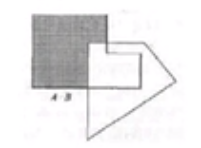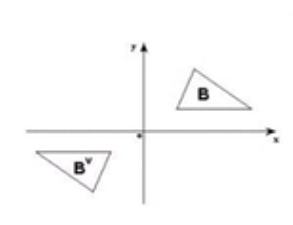$(A)_z=\{c|c=a+z,a \in A\}$

# 腐蚀与膨胀

## 膨胀

$A\oplus B=\{z|(\hat{B})_z \cap A \neq \Phi\}$
B称为结构元素。结构元素先进行反射，之后进行z平移，之后和A进行交运算，若击中，记录下平移量z。

$D_B(A)= A\oplus B=\{a|B_a\uparrow A\}$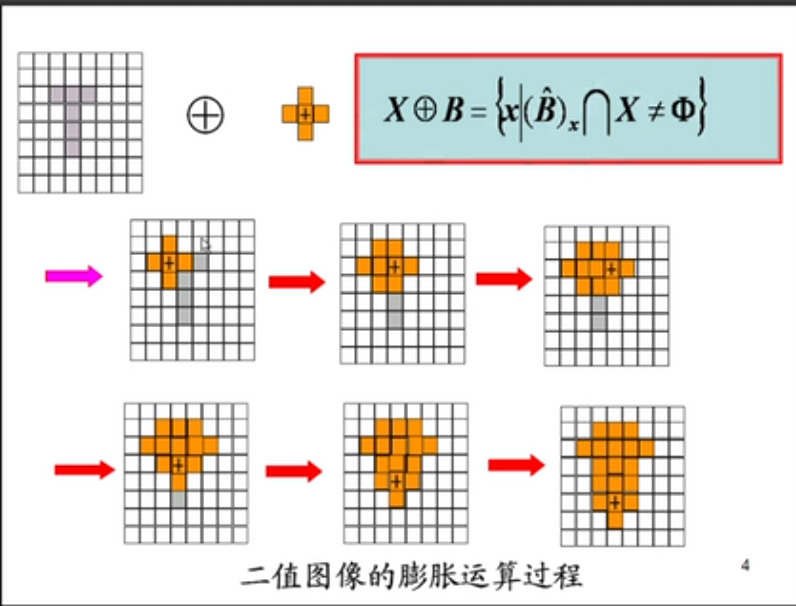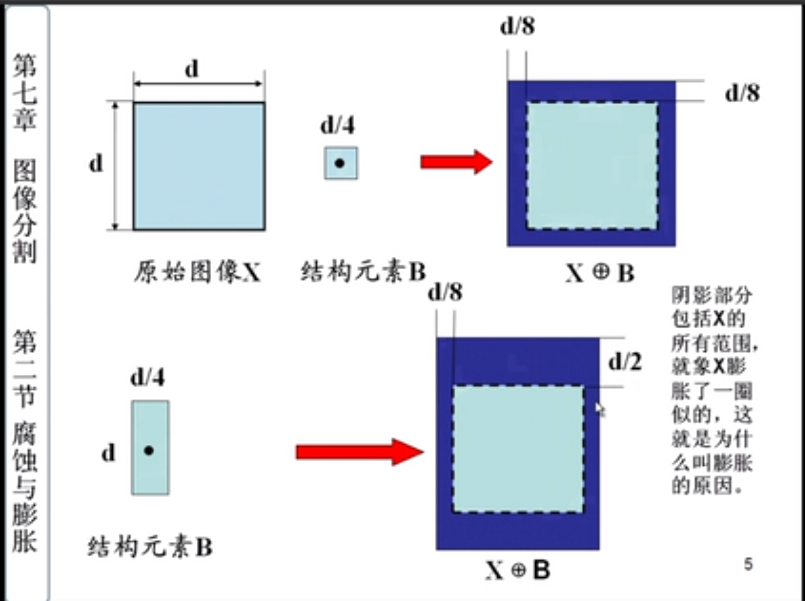## 腐蚀

$A\circleddash B=\{z|(B)_z \subseteq A\}$

$E_B(A)=A\circleddash B=\{a|B_a \subset A\}$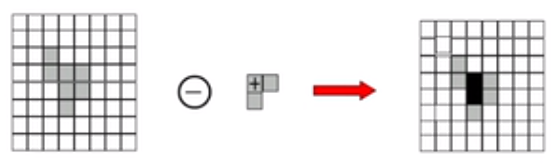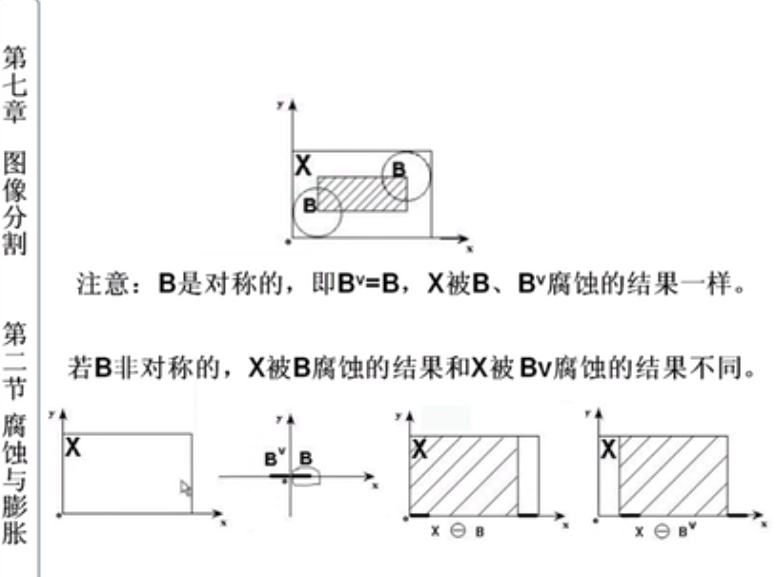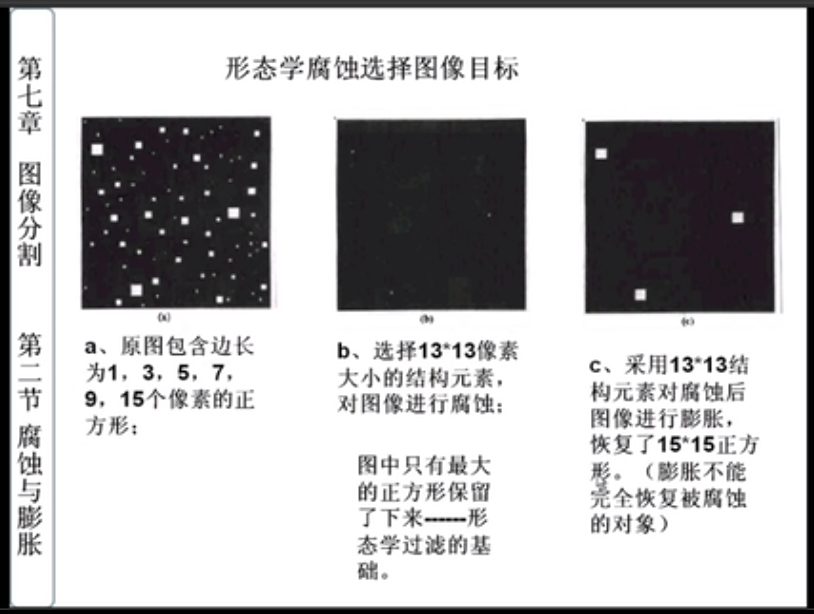## 膨胀和腐蚀的性质

• 膨胀和腐蚀运算的对偶性
$(X\circleddash B)^C=X^C \oplus \hat{B} \quad \quad (X\oplus B)^C=X^C \circleddash \hat{B}$
X对B的腐蚀运算的补集等于X的补集对B的反射的膨胀运算；
X对B的反射运算的补集等于X的补集对B的腐蚀的膨胀运算。
河岸的补集为河面，对河岸的腐蚀等价于对河面进行膨胀。

• 膨胀运算具有互换性，腐蚀运算不具有互换性。
$(X\oplus B)\oplus A=(X\oplus A)\oplus B$
$(X\circleddash B)\circleddash A \neq (X\circleddash A)\circleddash B$

• 腐蚀和膨胀具有组合性。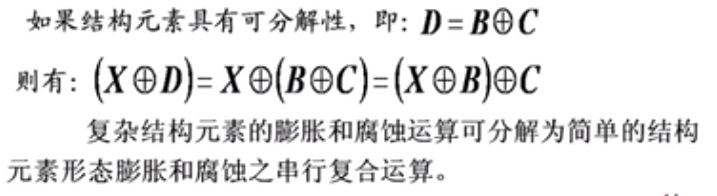# 开运算与闭运算

## 开运算

$A \circ B=(A\circleddash B)\oplus B$

• 消除细小对象
• 在细小粘连处分离对象
• 在不改变形状的前提下，平滑对象的边缘。

$3\times 3$$5\times 5$的矩形结构元素分别进行开运算：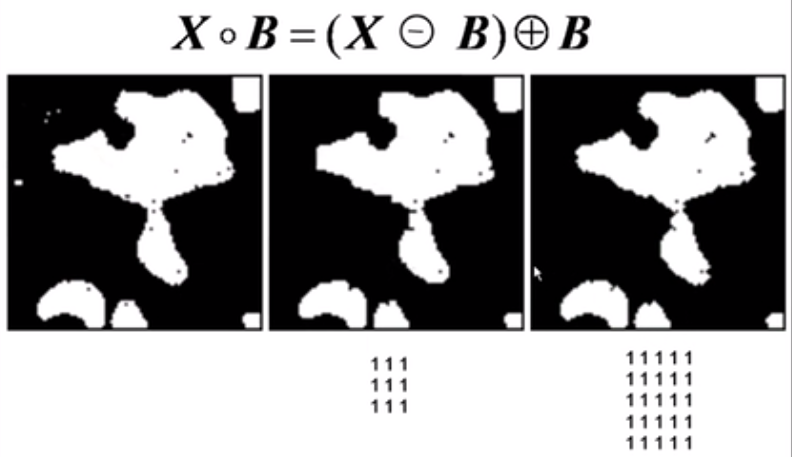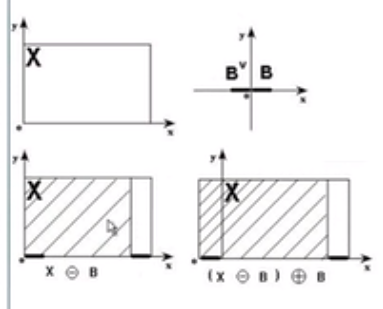## 闭运算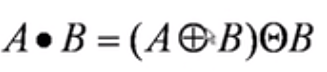• 填充对象内细小空洞
• 链接接近的对象
• 再不明显改变面积的前提下，平滑对象的边缘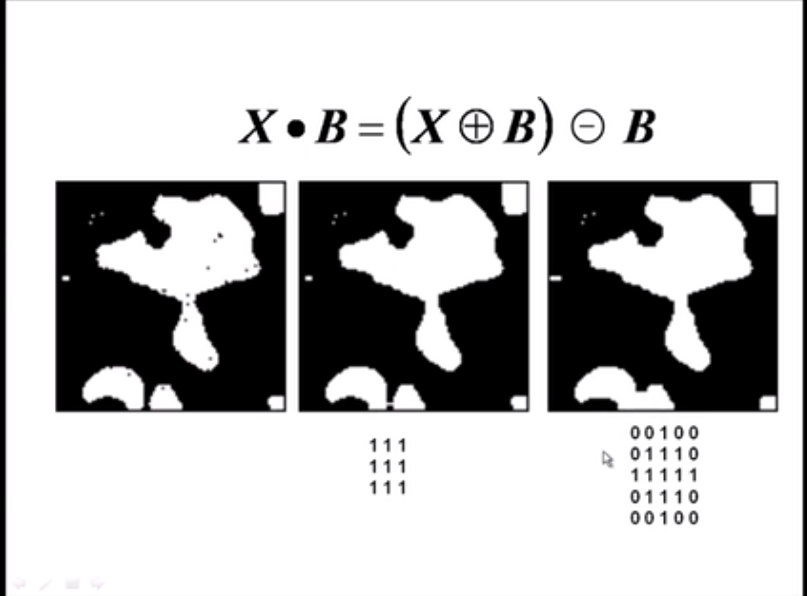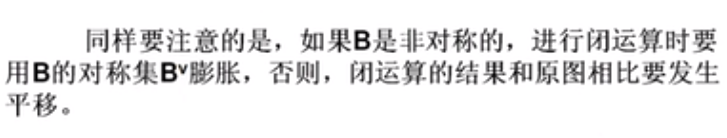## 开运算和闭运算的性质

X开运算的补集等于X补集的闭运算，或者X闭运算的补集等于X补集的开运算。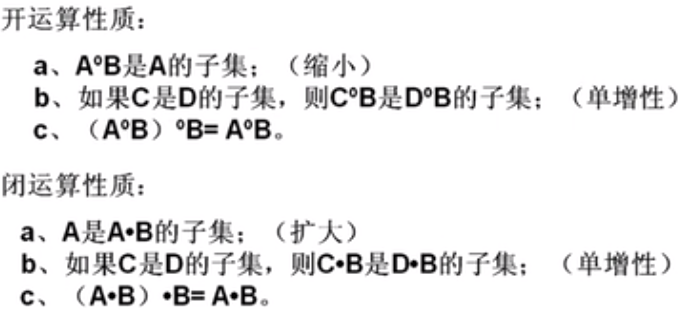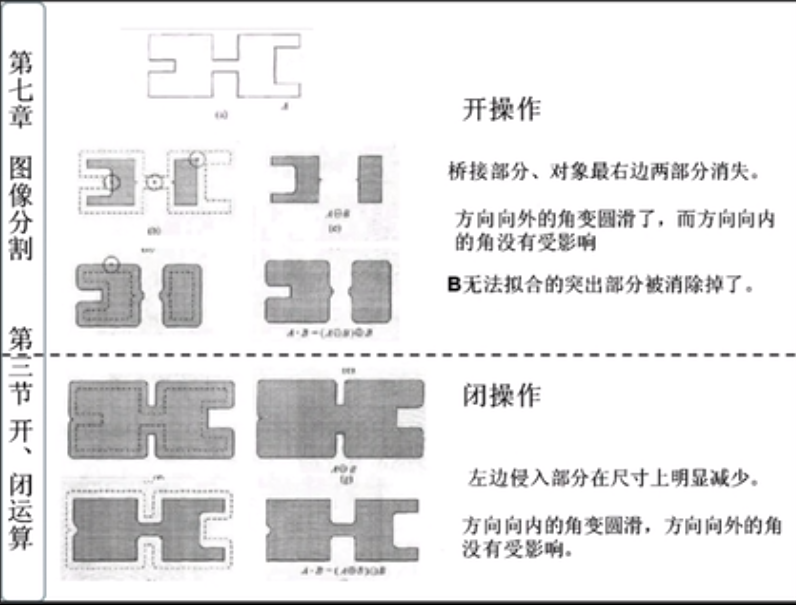# 二值数学形态学基本算法

## 形态滤波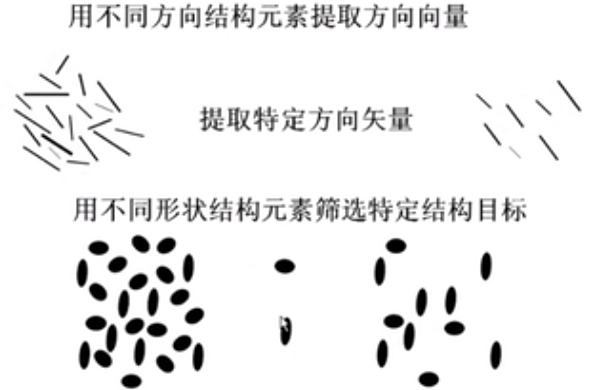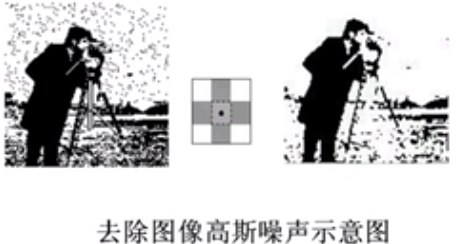## 图像的边缘提取

$Y=X-(X\circleddash B)$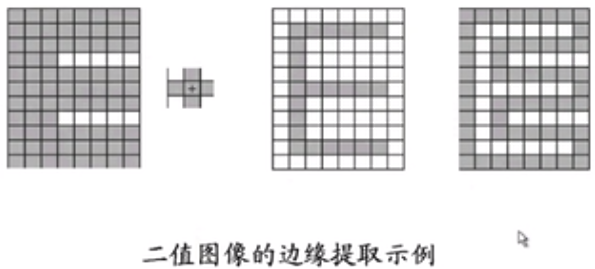## 区域填充

$X_k=(X_{k-1} \oplus B)\cap A^c$
B是结构元素，$X_0$是边界种子点，A是边界图像。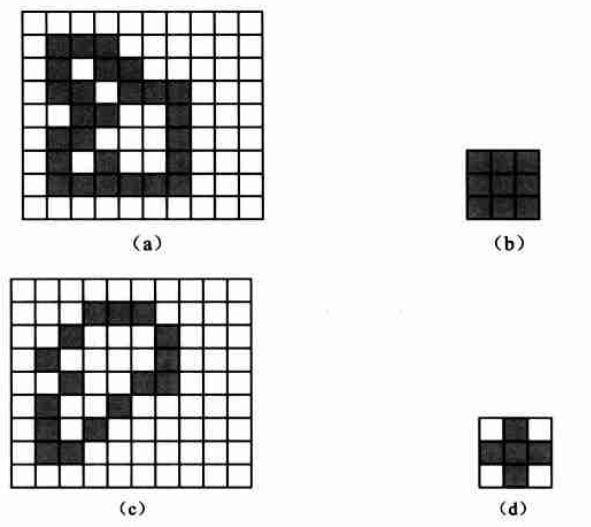## 击中击不中变换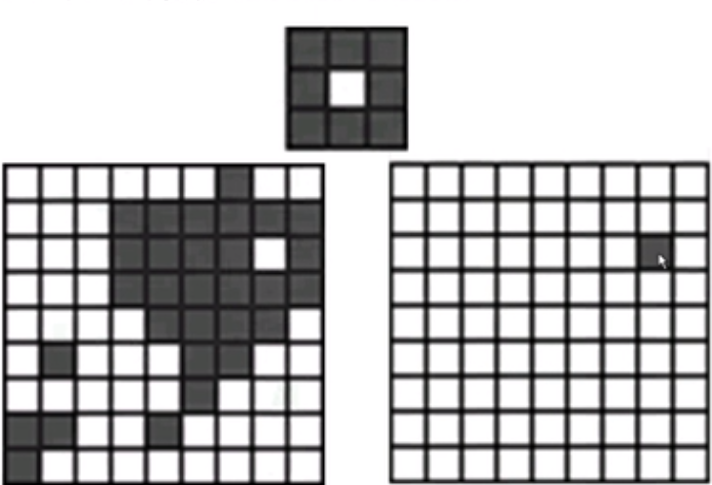2018-09-12 17:21:19 weixin_39880579 阅读数 818
• ###### MATLAB图像处理

全面系统的学习MATLAB在图像处理中的应用

19908 人正在学习 去看看 魏伟

4.1 图像数据表示的层次

4.2 传统图像数据结构

4.2.1 矩阵

• 二值图像（binary image）（仅有两个亮度级别的图像）用仅含有0和1的矩阵来表示。
• 多光谱图像（multispectral image)的信息可以用几个矩阵来表示，每个矩阵含有一个频带的图像。
• 分层图像数据结构（hierarchical image data structures)用不同分辨率的矩阵来获得。图像的这种分层表示对于具有处理阵列结构的并行计算机是非常方便的。

• 亮度直方图（brightness histogram）从概率的角度观察，标准化的直方图是如下现象的概率密度的估计：一个图像的像素具有某个亮度。
• 共生矩阵（co-occurrence matrix)是亮度为z的像素和亮度为y的像素的具有空间关系的两个像素的概率估计，可以用来描述纹理。
• 积分图像（integral image）的构造方式是位置处的值是原图像左上角所有像素的和：

4.2.2 链

4.2.3 拓扑数据结构

4.2.4 关系结构

4.3 分层数据结构

4.3.1 金字塔

M型金字塔是一个图像序列，其中是具有与原图像同样的分辨率和元素的图像，降低一半分辨率得到的图像。当创建金字塔3，通常只考虑维数是2的幂的方阵，这时则仅对应于一个像素。

4.3.2 四叉树

2017-03-06 10:55:21 u011430225 阅读数 1140
• ###### MATLAB图像处理

全面系统的学习MATLAB在图像处理中的应用

19908 人正在学习 去看看 魏伟

Opencv2.4.9源码分析——SimpleBlobDetector ： 代码注释比较详细

Convexity ， Inertia Ratio ，Circularity

# 图像分析：二值图像连通域标记  : 基于轮廓的标记

OpenCV】使用floodfill()实现PhotoShop魔棒功能 ： 使用给定颜色填充一个联通的区域

PS: Two-Pass 似乎无法解决一些凹的目标。

2017-05-03 10:11:50 qq_34784753 阅读数 7610
• ###### MATLAB图像处理

全面系统的学习MATLAB在图像处理中的应用

19908 人正在学习 去看看 魏伟

①进行图像复原时，我们可以借助先验的几何特征信息，利用形态学算子有效消除噪声，又可以保留图像的原有信息。

②进行边缘信息提取时，对噪声不敏感，能够得到光滑的边缘结果

③对图像进行骨架提取时，也能够得到比较连续的结果

④算法便于使用硬件进行实现

1.集合运算

p ∈ A

(a.并集，交集，差集和补集

（1.并集：A∪B = {p| p∈A or p∈B }

A∪B 中的元素属于A或是属于B

（2.交集：A∩B = {p| p∈A and p∈B }

A∪B 中的元素既属于A也属于B

（3.补集：不在集合A中的元素构成A的补集（4.差集：A-B 即为在集合A中，但是不在集合B中的元素(b.平移和映射

Â = {p | p = -q，q ∈ A}

(A)z = {p | p = q+z，q ∈ A}

(A)z包含的元素为集合A中的每一个坐标与位移相加而形成的新坐标。

2.二值图像的逻辑运算

3.结构元素

4.二值图像的形态学基本运算

（1.膨胀操作（①将结构元素B关于原点的映射(B)平移z

（②几个A与结构元素B的映射平移(B)z的交集不为空集

B对A膨胀生成的集合是B的映射平移集合与集合A至少有1个非0元素时B的原点位置的集合

→对于原点属于结构元素的膨胀运算，总是成立

→对于一幅二值图像，膨胀运算可以扩张白色目标区域，填补目标区域中尺寸小于结构元素的孔洞和缺口。

（2.腐蚀操作→将节后元素B平移后仍完全包含在集合A中

→B对A腐蚀生成的集合是B的平移集合完全包含在集合A中B的原点位置的集合。

→对于原点属于结构元素的腐蚀运算,总是成立

→对于原点不属于结构元素的腐蚀运算，不一定成立

→对于一幅二值图像，腐蚀运算可以收缩白色的目标区域，消除了储存小于结构元素的目标和毛刺。

（3.膨胀和腐蚀操作的对偶性→对图像中目标区域的膨胀运算相当于对背景区域的腐蚀，反之亦然

→图像的膨胀与腐蚀是一对互为对偶的运算操作→整个二值图像形态学体系建立在一个基本运算的基础之上。

5.使用OpenCV实现膨胀和腐蚀

void erode( InputArray src, OutputArray dst, InputArray kernel,Point anchor=Point(-1,-1), int iterations=1,
int borderType=BORDER_CONSTANT,const Scalar& borderValue=morphologyDefaultBorderValue() );

void dilate( InputArray src, OutputArray dst, InputArray kernel,Point anchor=Point(-1,-1), int iterations=1,
int borderType=BORDER_CONSTANT, const Scalar& borderValue=morphologyDefaultBorderValue() );

→通常，我们一般使用函数getStructuringElement配合这个参数的使用。getStructuringElement函数会返回指定形状和尺寸的结构元素(内核矩阵)。其中，getStructuringElement

·矩形：MORPH_RECT;

·交叉形：MORPH_CROSS;

·椭圆形：MORPH_ELLIPSE;

getStructuringElement函数的第二和第三个参数分别是内核的尺寸以及锚点的位置。

//获取自定义核
Mat element = getStructuringElement(MORPH_RECT,Size(15,15));

#include <iostream>
#include <opencv2\core\core.hpp>
#include <opencv2\highgui\highgui.hpp>
#include <opencv2\imgproc\imgproc.hpp>

using namespace cv;
using namespace std;

int main()
{
if (!srcImage.data)
{
cout << "读入图片错误" << endl;
system("pause");
return -1;
}
Mat srcBinary;
threshold(srcImage, srcBinary, 0, 255, THRESH_OTSU);
imshow("原始图像", srcBinary);
//获取自定义核
Mat element = getStructuringElement(MORPH_RECT, Size(15, 15));
Mat ErodeImage;
Mat DilateImage;
erode(srcBinary, ErodeImage, element);
imshow("腐蚀效果图", ErodeImage);
dilate(srcBinary, DilateImage, element);
imshow("膨胀效果图", DilateImage);
waitKey();
return 0;
}6.开运算与闭运算

（1.开运算→开运算可以消除小尺寸的目标和细小的突出部分，断开细长的桥接部分从而分离出目标区域，并在不明显改变目标区域面积的条件下平滑较大目标边界的作用。

→设有结构元素B和集合A，则开运算的实质就是让B在A的区域内部全部平移，可以表示为（2.闭运算→闭运算可以填补目标区域内部小尺寸孔洞和细窄的缺口，桥接狭窄的断裂部分从而使目标区域变得连通，并且可以再不明显改变目标区域面积的条件下平滑较大目标边界的作用。

→从几何意义上讲，对于结构元素B，当且仅当包括元素p的任何平移集合(B)z∩A≠∅时，p属于集合A•B

→图示如下：（3.开运算与闭运算的对偶性

→开运算与闭运算也同样具有对偶性，可以表示如下：→开运算与闭运算的结合同时具有开运算和闭运算的作用

→开运算具有消除尺寸小于结构元素的孤立目标和突起的作用

→闭运算填补了目标区域内部尺寸小于节后元素的孔洞和缺口

→开运算与闭运算的结合经常用于二值图像的后处理阶段，整个过程消除了二值图像中的孤立目标，并且填补了目标区域的内部孔洞。

→但是这两种运算会破坏目标原本轮廓和形状，特别是对于小尺寸目标会变得十分明显。

（4.使用OpenCV实现开运算和闭运算

void morphologyEx( InputArray src, OutputArray dst,int op, InputArray kernel,Point anchor=Point(-1,-1), int iterations=1,
int borderType=BORDER_CONSTANT,const Scalar& borderValue=morphologyDefaultBorderValue() );//实现形态学开运算和闭运算
#include <iostream>
#include <opencv2\core\core.hpp>
#include <opencv2\highgui\highgui.hpp>
#include <opencv2\imgproc\imgproc.hpp>

using namespace cv;
using namespace std;

int main()
{
Mat BinaryImage;
threshold(srcImage, BinaryImage, 0, 255, THRESH_OTSU);
imshow("原图像", BinaryImage);
//定义结构元素
Mat element = getStructuringElement(MORPH_ELLIPSE, Size(15, 15));
//形态学闭运算
Mat closeImage;
morphologyEx(BinaryImage, closeImage, MORPH_CLOSE, element);
imshow("闭运算操作", closeImage);
//形态学开运算
Mat openImage;
morphologyEx(BinaryImage, openImage, MORPH_OPEN, element);
imshow("开运算操作", openImage);
waitKey();
return 0;
}